# Texas Go Math Grade 1 Lesson 10.6 Answer Key Count by Tens to 120

Refer to our Texas Go Math Grade 1 Answer Key Pdf to score good marks in the exams. Test yourself by practicing the problems from Texas Go Math Grade 1 Lesson 10.6 Answer Key Count by Tens to 120.

## Texas Go Math Grade 1 Lesson 10.6 Answer Key Count by Tens to 120

Explore

Start on 10. Count forward by tens. Color each number as you say it.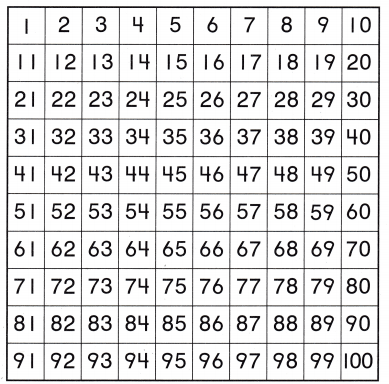In math, to count can be defined as the act of determining the quantity or the total number of objects in a set or a group.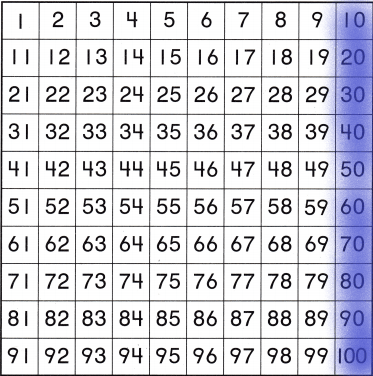Explanation:
The number we need to start from 10. From there we need to add 10s. By using the skip count by 10 technique we should add 10.
By math sentence,
10+10=20
20+10=30
30+10=40
40+10=50
50+10=60
60+10=70
70+10=80
80+10=90
90+10=100 and so on… so I coloured the last column in the counting track.

FOR THE TEACHER • Read the following problem. When counting by tens, what number comes after 40?
50 will come after 40 when we continue adding 10. I represented n the below diagram.Math Talk
Mathematical Processes

Which numbers the hundred chart, did you colour? Explain.
A hundred board is one of the best math resources you can have available for your child. It can help with counting, number recognition, adding and subtracting, and even discovering number patterns.
Here, I coloured the last column in the counting chart because the question asked to start from 10 and count the numbers by using skip count by 10.
The numbers I coloured was 10, 20, 30, 40, 50, 60, 70, 80, 90, 100.
Adding 10 to every number we get the next number.

Model and Draw

Start on 3. Count by tens. Write the numbers.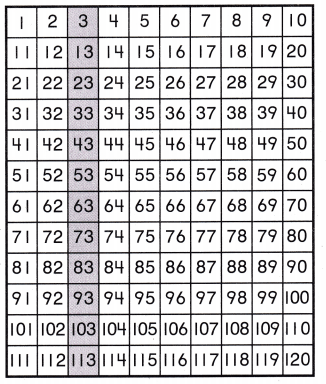THINK: When you count by tens, each number is ten more.
3, 13, 23, 33, ______, ______, ______, ______, ______, ______, ______, ______
When we skip by10 in between while counting the numbers, then it is said to skip counting by 10. Skip count by 10 is very easy and quick to do. See the below-given example to understand it better.
The question asked was to start from 3 then we add 10 to every number.
3+10=13
13+10=23
23+10=33
33+10=43
43+10=53
53+10=63
63+10=73
73+10=83
83+10=93 and so on… continues.
The numbers are 3, 13, 23, 33, 43, 53, 63, 73, 83, 93, 103, 113.

Share and Show

Use a Counting Chart to count by tens. Write the numbers.

Question 1.
Start on 17.
17, ______, ______, ______, ______, ______, ______, ______, ______
When we skip by10 in between while counting the numbers, then it is said to skip counting by 10. Skip count by 10 is very easy and quick to do. See the below-given example to understand it better.
1. Skip counting in ten means to keep adding ten to a number.
2. A one hundred number grid is a useful way to teach skip counting.
3. Every time we add 10, we move one square down the number grid.
4. We count up in tens from 17, adding 10 each time.
5. We have 17, 27, 37, 47, 57, 67, 77, 87, and 97.
6. When skip counting in tens, the numbers will all end in the same digit.
7. The digit in the tens column will increase by one each time.
The question asked was to start from 17 then we add 10 to every number.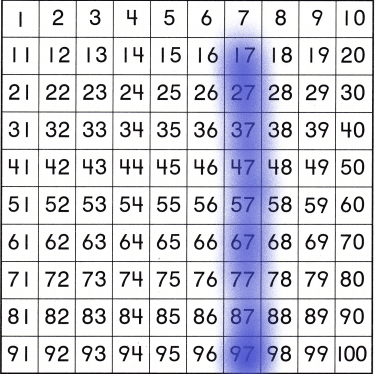17+10=27
27+10=37
37+10=47
47+10=57 and so on…

Question 2.
Start on 1.
1, ______, ______, ______, ______, ______, ______, ______, ______
When we skip by10 in between while counting the numbers, then it is said to skip counting by 10. Skip count by 10 is very easy and quick to do. See the below-given example to understand it better.
1. Skip counting in ten means to keep adding ten to a number.
2. A one hundred number grid is a useful way to teach skip counting.
3. Every time we add 10, we move one square down the number grid.
4. We count up in tens from 1, adding 10 each time.
5. We have 1, 11, 21, 31, 41, 51, 61, 71, 81, and 91.
6. When skip counting in tens, the numbers will all end in the same digit.
7. The digit in the tens column will increase by one each time.
The question asked was to start from 1 then we add 10 to every number.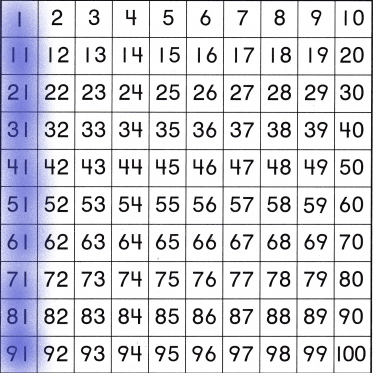1+10=11
11+10=21
21+10=31
31+10=41
41+10=51 and so on…

Question 3.
Start on 39.
39, ______, ______, ______, ______, ______, ______, ______, ______
When we skip by10 in between while counting the numbers, then it is said to skip counting by 10. Skip count by 10 is very easy and quick to do. See the below-given example to understand it better.
1. Skip counting in ten means to keep adding ten to a number.
2. A one hundred number grid is a useful way to teach skip counting.
3. Every time we add 10, we move one square down the number grid.
4. We count up in tens 39, adding 10 each time.
5. We have 39, 49, 59, 69, 79, 89, 99, 109, and 119.
6. When skip counting in tens, the numbers will all end in the same digit.
7. The digit in the tens column will increase by one each time.
The question asked was to start from 39 then we add 10 to every number.39+10=49
49+10=59
59+10=69
69+10=79
79+10=89
89+10=99 and so on…

Problem Solving

Use a Counting Chart. Count by tens. Write the numbers.

Question 4.
40, ______, ______, ______, ______, ______, ______, ______, ______
When we skip by10 in between while counting the numbers, then it is said to skip counting by 10. Skip count by 10 is very easy and quick to do. See the below-given example to understand it better.
1. Skip counting in ten means to keep adding ten to a number.
2. A one hundred number grid is a useful way to teach skip counting.
3. Every time we add 10, we move one square down the number grid.
4. We count up in tens 40, adding 10 each time.
5. We have 40, 50, 60, 70, 80, 90, 100, 110, and 120.
6. When skip counting in tens, the numbers will all end in the same digit.
7. The digit in the tens column will increase by one each time.
The question asked was to start from 40 then we add 10 to every number.40+10=50
50+10=60
60+10=70
70+10=80
80+10=90 and so on…

Question 5.
15, ______, ______, ______, ______, ______, ______, ______, ______
When we skip by10 in between while counting the numbers, then it is said to skip counting by 10. Skip count by 10 is very easy and quick to do. See the below-given example to understand it better.
1. Skip counting in ten means to keep adding ten to a number.
2. A one hundred number grid is a useful way to teach skip counting.
3. Every time we add 10, we move one square down the number grid.
4. We count up in tens 15, adding 10 each time.
5. We have 15, 25, 35, 45, 55, 65, 75, 85, 95.
6. When skip counting in tens, the numbers will all end in the same digit.
7. The digit in the tens column will increase by one each time.
The question asked was to start from 15 then we add 10 to every number.15+10=25
25+10=35
35+10=45
45+10=55
55+10=65 and so on…

Question 6.
28, ______, ______, ______, ______, ______, ______, ______, ______
When we skip by10 in between while counting the numbers, then it is said to skip counting by 10. Skip count by 10 is very easy and quick to do. See the below-given example to understand it better.
1. Skip counting in ten means to keep adding ten to a number.
2. A one hundred number grid is a useful way to teach skip counting.
3. Every time we add 10, we move one square down the number grid.
4. We count up in tens 28, adding 10 each time.
5. We have 28, 38, 48, 58, 68, 78, 88, 98, 108, and 118.
6. When skip counting in tens, the numbers will all end in the same digit.
7. The digit in the tens column will increase by one each time.
The question asked was to start from 28 then we add 10 to every number.28+10=38
38+10=48
48+10=58
58+10=68
68+10=78 and so on…

Question 7.
6, ______, ______, ______, ______, ______, ______, ______, ______
When we skip by10 in between while counting the numbers, then it is said to skip counting by 10. Skip count by 10 is very easy and quick to do. See the below-given example to understand it better.
1. Skip counting in ten means to keep adding ten to a number.
2. A one hundred number grid is a useful way to teach skip counting.
3. Every time we add 10, we move one square down the number grid.
4. We count up in tens 6, adding 10 each time.
5. We have 6, 16, 26, 36, 46, 56, 66, 76, 86.
6. When skip counting in tens, the numbers will all end in the same digit.
7. The digit in the tens column will increase by one each time.
The question asked was to start from 6 then we add 10 to every number.6+10=16
16+10=26
26+10=36
36+10=46
46+10=56 and so on…

Question 8.
14, ______, ______, ______, ______, ______, ______, ______, ______
When we skip by10 in between while counting the numbers, then it is said to skip counting by 10. Skip count by 10 is very easy and quick to do. See the below-given example to understand it better.
1. Skip counting in ten means to keep adding ten to a number.
2. A one hundred number grid is a useful way to teach skip counting.
3. Every time we add 10, we move one square down the number grid.
4. We count up in tens 14, adding 10 each time.
5. We have 14, 24, 34, 44, 54, 64, 74, 84, 94.
6. When skip counting in tens, the numbers will all end in the same digit.
7. The digit in the tens column will increase by one each time.
The question asked was to start from 14 then we add 10 to every number.14+10=24
24+10=34
34+10=44
44+10=54
54+10=64 and so on…

Question 9.
32, ______, ______, ______, ______, ______, ______, ______, ______
When we skip by10 in between while counting the numbers, then it is said to skip counting by 10. Skip count by 10 is very easy and quick to do. See the below-given example to understand it better.
1. Skip counting in ten means to keep adding ten to a number.
2. A one hundred number grid is a useful way to teach skip counting.
3. Every time we add 10, we move one square down the number grid.
4. We count up in tens 32, adding 10 each time.
5. We have 32, 42, 52, 62, 72, 82, 92, 102, and 112.
6. When skip counting in tens, the numbers will all end in the same digit.
7. The digit in the tens column will increase by one each time.
The question asked was to start from 32 then we add 10 to every number.32+10=42
42+10=52
52+10=62
62+10=72
72+10=82 and so on…

Solve.

Question 10.
If you start on 43 and count by tens, what number is after 73 and before 93?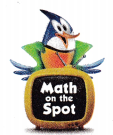Explanation:
When we skip by10 in between while counting the numbers, then it is said to skip counting by 10. Skip count by 10 is very easy and quick to do. See the below-given example to understand it better.
1. Skip counting in ten means to keep adding ten to a number.
2. A one hundred number grid is a useful way to teach skip counting.
3. Every time we add 10, we move one square down the number grid.
4. We count up in tens 43, adding 10 each time.
5. We have 43, 53, 63, 73, 83, 93.
6. When skip counting in tens, the numbers will all end in the same digit.
7. The digit in the tens column will increase by one each time.
The question asked were we start at 43 and count by 10s. The number we need to find is after 73 and before 93.
The number 83 will come after 73 and before 93.Question 11.
H.O.T. Multi-Step You say me when you start on 21 and count by tens. I am after 91. I am before 111. What number am I?
Explanation:
When we skip by10 in between while counting the numbers, then it is said to skip counting by 10. Skip count by 10 is very easy and quick to do. See the below-given example to understand it better.
1. Skip counting in ten means to keep adding ten to a number.
2. A one hundred number grid is a useful way to teach skip counting.
3. Every time we add 10, we move one square down the number grid.
4. We count up in tens 21, adding 10 each time.
5. We have 21, 31, 41, 51, 61, 71, 81, 91, 101, 111.
6. When skip counting in tens, the numbers will all end in the same digit.
7. The digit in the tens column will increase by one each time.
The question asked were we start at 21 and count by 10s. The number we need to find is after 91 and before 111.
The number 101 will come after 91 and before 111.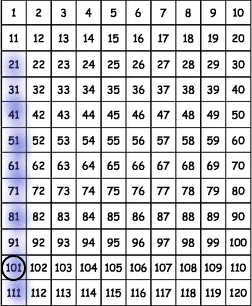Question 12.
Analyze The giraffe eats 84 leaves. Then she eats 10 leaves off one tree and 10 off another. Count forward by tens. How many leaves does she eat?
(A) 104
(B) 70
(C) 94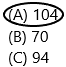Explanation
The total number of leaves giraffe eats=84
The number of leaves she eats on one tree=10
The number of leaves she leaves on another tree=10
The total number of leaves the giraffe eats=X
Now add all the leaves she eats: 84+10+10=104.
The value of X is 104.

Question 13.
Gavin can count 10 numbers at a time. He starts at 6 and counts 11 times. Count forward by tens. What number does Gavin land on?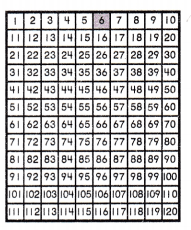(A) 96
(B) 116
(C) 17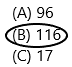Explanation:
When we skip by10 in between while counting the numbers, then it is said to skip counting by 10. Skip count by 10 is very easy and quick to do. See the below-given example to understand it better.
1. Skip counting in ten means to keep adding ten to a number.
2. A one hundred number grid is a useful way to teach skip counting.
3. Every time we add 10, we move one square down the number grid.
4. We count up in tens 6, adding 10 each time.
5. We have 6, 16, 26, 36, 46, 56, 66, 76, 86, 96, 106 and 116.
6. When skip counting in tens, the numbers will all end in the same digit.
7. The digit in the tens column will increase by one each time.
The question asked were we start at 6 and counted 11 times by using skip count by 10s.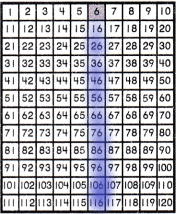Counted 11 times means count from 16, 26, 36, 46, 56, 66, 76, 86, 96, 106, 116.

Question 14.
Multi-Step Kay’s fish tank only holds 120 fish. She adds 10 fish once a week. She does this for 2 weeks. How many more weeks will it take to fill the tank?
(A) 12
(B) 10
(C) 8Explanation:
The total number of fishes in the tank=120
The number of fishes she adds in a tank once a week=10
The number of weeks she added 10 fishes in the tank=2
We need to calculate the number of weeks she used to take to add all the fish in the tank.
The number of weeks=X
X=100/10
X=10.
It means she takes 10 more weeks to add the fish.

Question 15.
Texas Test Prep Count by tens. What numbers complete the count by tens sequence?
3, 13, 23, ________, 53
(A) 24, 25
(B) 33, 43
(C) 63, 73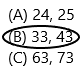Explanation:
1. Skip counting in ten means to keep adding ten to a number.
2. A one hundred number grid is a useful way to teach skip counting.
3. Every time we add 10, we move one square down the number grid.
4. We count up in tens 3, adding 10 each time.
5. We have 3, 13, 23, 33, 43, 53.
6. When skip counting in tens, the numbers will all end in the same digit.
7. The digit in the tens column will increase by one each time.

TAKE HOME ACTIVITY • Write these numbers: 2. 12, 22, 32. 42. Ask your child to tell you the next 5 numbers.
Explanation: The next five numbers are 52, 62, 72, 82, 92.
1. Skip counting in ten means to keep adding ten to a number.
2. A one hundred number grid is a useful way to teach skip counting.
3. Every time we add 10, we move one square down the number grid.
4. We count up in tens 2, adding 10 each time.
5. We have 2, 12, 22, 32, 42, 52, 62, 72, 82, 92.
6. When skip counting in tens, the numbers will all end in the same digit.
7. The digit in the tens column will increase by one each time.

### Texas Go Math Grade 1 Lesson 10.6 Homework and Practice Answer Key

Count by tens. Write the numbers.

Question 1.
Start on 2.
2, ______, ______, ______, ______, ______, ______, ______, ______
When we skip by10 in between while counting the numbers, then it is said to skip counting by 10. Skip count by 10 is very easy and quick to do. See the below-given example to understand it better.
1. Skip counting in ten means to keep adding ten to a number.
2. A one hundred number grid is a useful way to teach skip counting.
3. Every time we add 10, we move one square down the number grid.
4. We count up in tens 2, adding 10 each time.
5. We have 2, 12, 22, 32, 42, 52, 62, 72, and 82.
6. When skip counting in tens, the numbers will all end in the same digit.
7. The digit in the tens column will increase by one each time.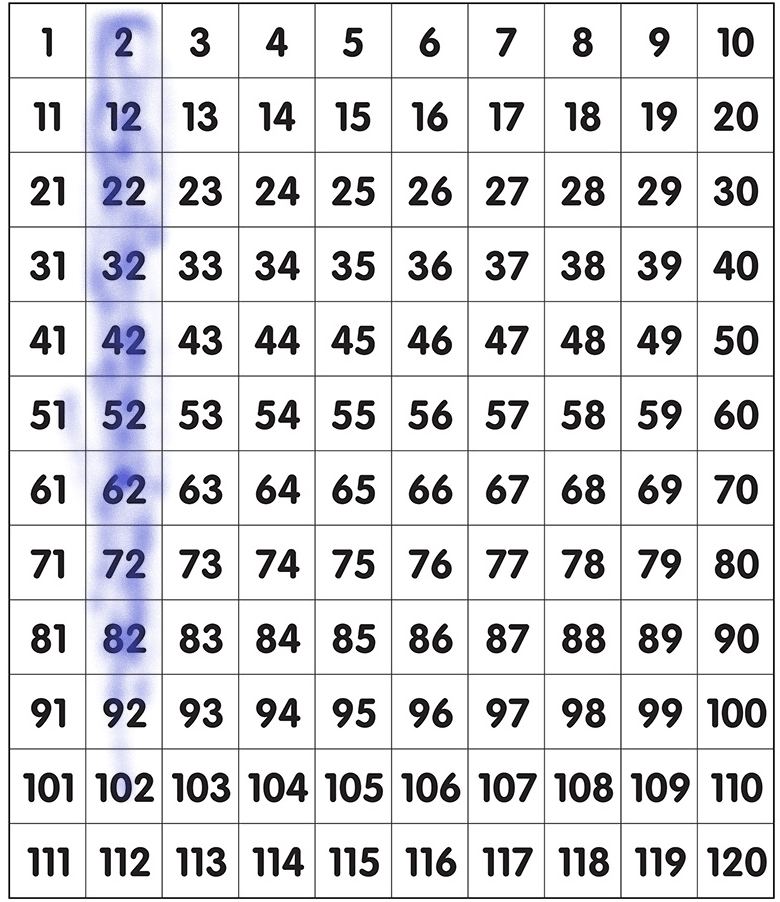2+10=12
12+10=22
22+10=32
32+10=42
42+10=52 and so on…

Question 2.
Start on 35.
35, ______, ______, ______, ______, ______, ______, ______, ______
When we skip by10 in between while counting the numbers, then it is said to skip counting by 10. Skip count by 10 is very easy and quick to do. See the below-given example to understand it better.
1. Skip counting in ten means to keep adding ten to a number.
2. A one hundred number grid is a useful way to teach skip counting.
3. Every time we add 10, we move one square down the number grid.
4. We count up in tens 35, adding 10 each time.
5. We have 35, 45, 55, 65, 75, 85, 95, 105, 115.
6. When skip counting in tens, the numbers will all end in the same digit.
7. The digit in the tens column will increase by one each time.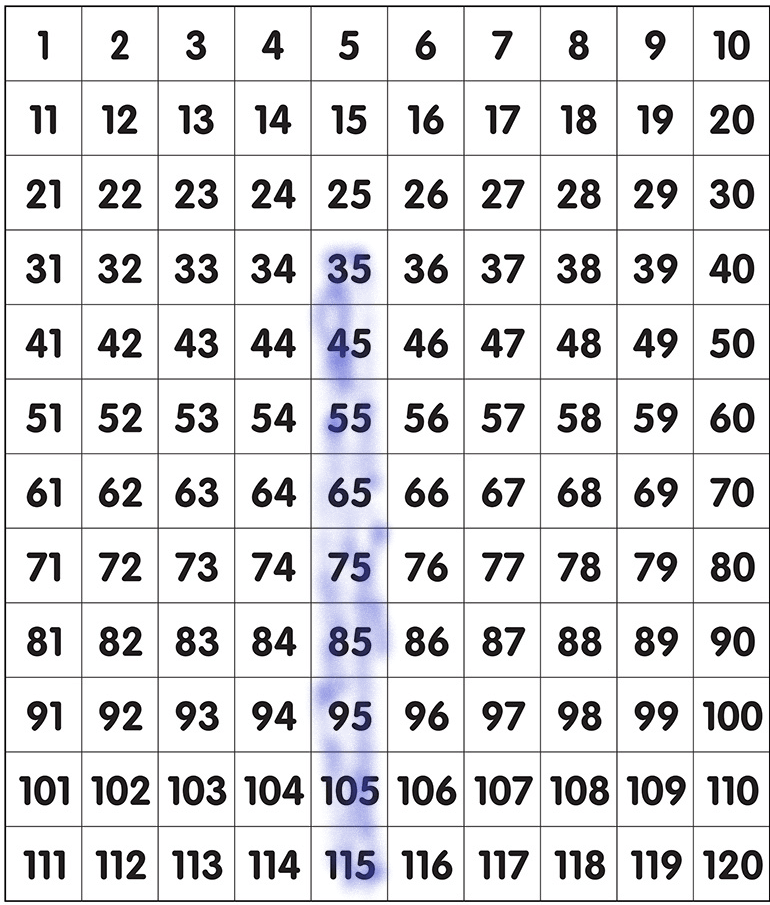35+10=45
45+10=55
55+10=65
65+10=75 and so on…

Question 3.
Start on 27.
27, ______, ______, ______, ______, ______, ______, ______, ______
When we skip by10 in between while counting the numbers, then it is said to skip counting by 10. Skip count by 10 is very easy and quick to do. See the below-given example to understand it better.
1. Skip counting in ten means to keep adding ten to a number.
2. A one hundred number grid is a useful way to teach skip counting.
3. Every time we add 10, we move one square down the number grid.
4. We count up in tens 27, adding 10 each time.
5. We have 27, 37, 47, 57, 67, 77, 87, 97, 107, 117.
6. When skip counting in tens, the numbers will all end in the same digit.
7. The digit in the tens column will increase by one each time.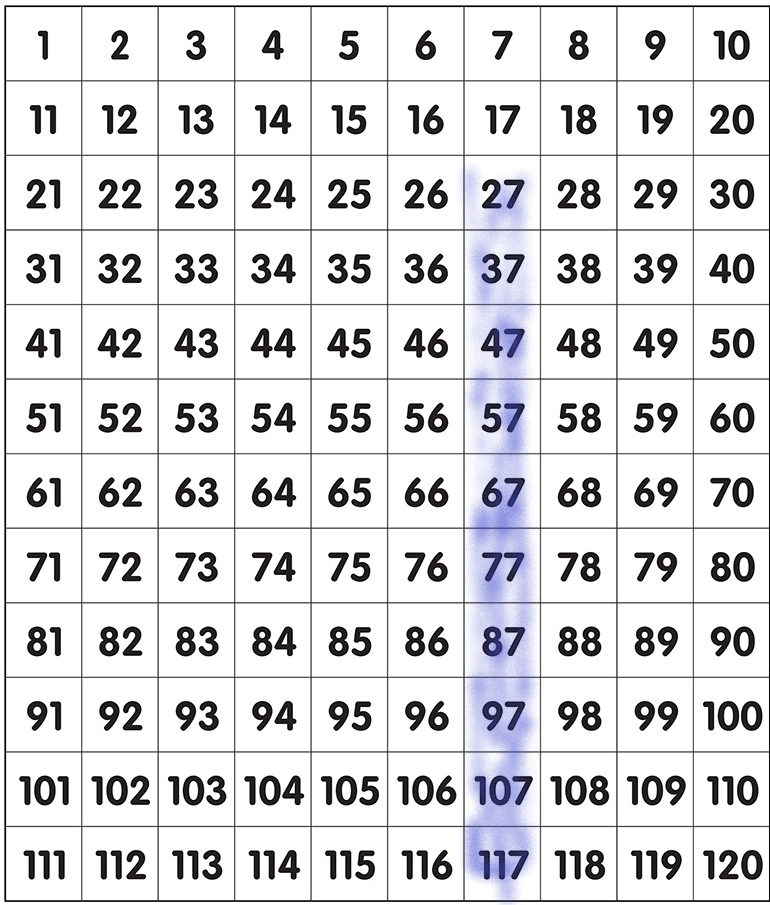27+10=37
37+10=47
47+10=57
57+10=67
67+10=77 and so on…

Question 4.
Start on 13.
13, ______, ______, ______, ______, ______, ______, ______, ______
When we skip by10 in between while counting the numbers, then it is said to skip counting by 10. Skip count by 10 is very easy and quick to do. See the below-given example to understand it better.
1. Skip counting in ten means to keep adding ten to a number.
2. A one hundred number grid is a useful way to teach skip counting.
3. Every time we add 10, we move one square down the number grid.
4. We count up in tens 13, adding 10 each time.
5. We have 13, 23, 33, 43, 53, 63, 73, 83, 93.
6. When skip counting in tens, the numbers will all end in the same digit.
7. The digit in the tens column will increase by one each time.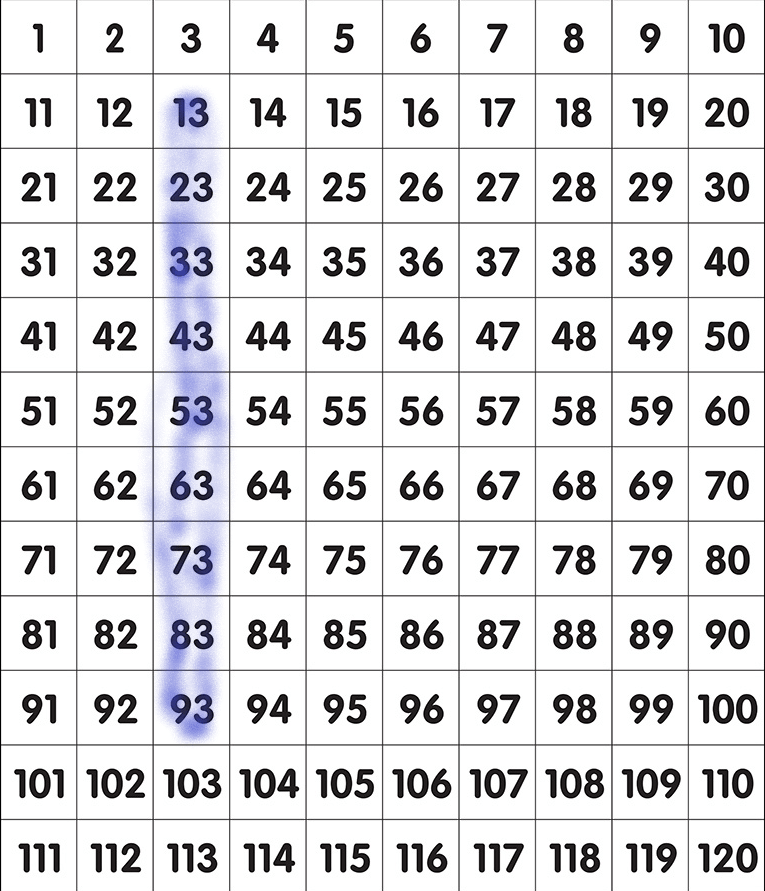13+10=23
23+10=33
33+10=43
43+10=53 and so on…

Problem Solving

Solve.

Question 5.
Libby has 49 pennies. She saves 10 pennies a week for the next 6 weeks. Count forward by tens. How many pennies does Libby have now?
__________ pennies
The total number of pennies=49
The number of pennies she saves in a week=10
The number of more weeks she saves=6
The total number of pennies does Libby have for the next 6 weeks=X.
use count by 10 technique.
49+10=59 (first week)
59+10=69 (second week)
69+10=79 (third week)
79+10=89 (fourth week)
89+10=99 (fifth week)
99+10=109 (sixth week).

Lesson Check

Question 6.
Rodney feeds the rabbits at the petting zoo. He gives them 19 carrots. Then he gives them 10 more carrots. How many carrots did Rodney give the rabbits?(A) 29
(B) 19
(C) 21Explanation:
The number of carrots she gives to rabbit=19
The number of carrots adds more=10
The total number of carrots=19+10=29.

Question 7.
Shannon has 54 shells. She collects some more. She counts 5 more sets of 10 shells. How many shells does Shannon have now?(A) 64
(B) 74
(C) 104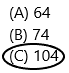Explanation:
The number of shells Shannon has=54
The number of sets she count=5
The number of shells she counts extra=10
The number of the shells she has after counting 5 more extra=10*5=50.
The total number of shells Shannon has=50+54=104.

Question 8.
Multi-Step Alan needs 100 dimes to fill his coin collection book. Each page holds 10 dimes. He fills 2 pages. How many more pages does he need to fill the book?
(A) 10
(B) 8
(C) 2Explanation:
The total number of dimes Alan needs to fill the book=100
The number of the pages were already filled=2
The number of dimes per page=10
The total number of dimes he already filled in the book=10*2=20.
Now we need to calculate the number of dimes needed more to fill the complete book.
The number of dimes=100-20=80.
Therefore, 80 dimes are needed to fill the collection book.

Question 9.
Count by tens. What numbers are not shown?
6, 16, 26, ______, _______, ______, 66
(A) 36, 46, 56
(B) 27, 28, 29
(C) 30, 40, 50